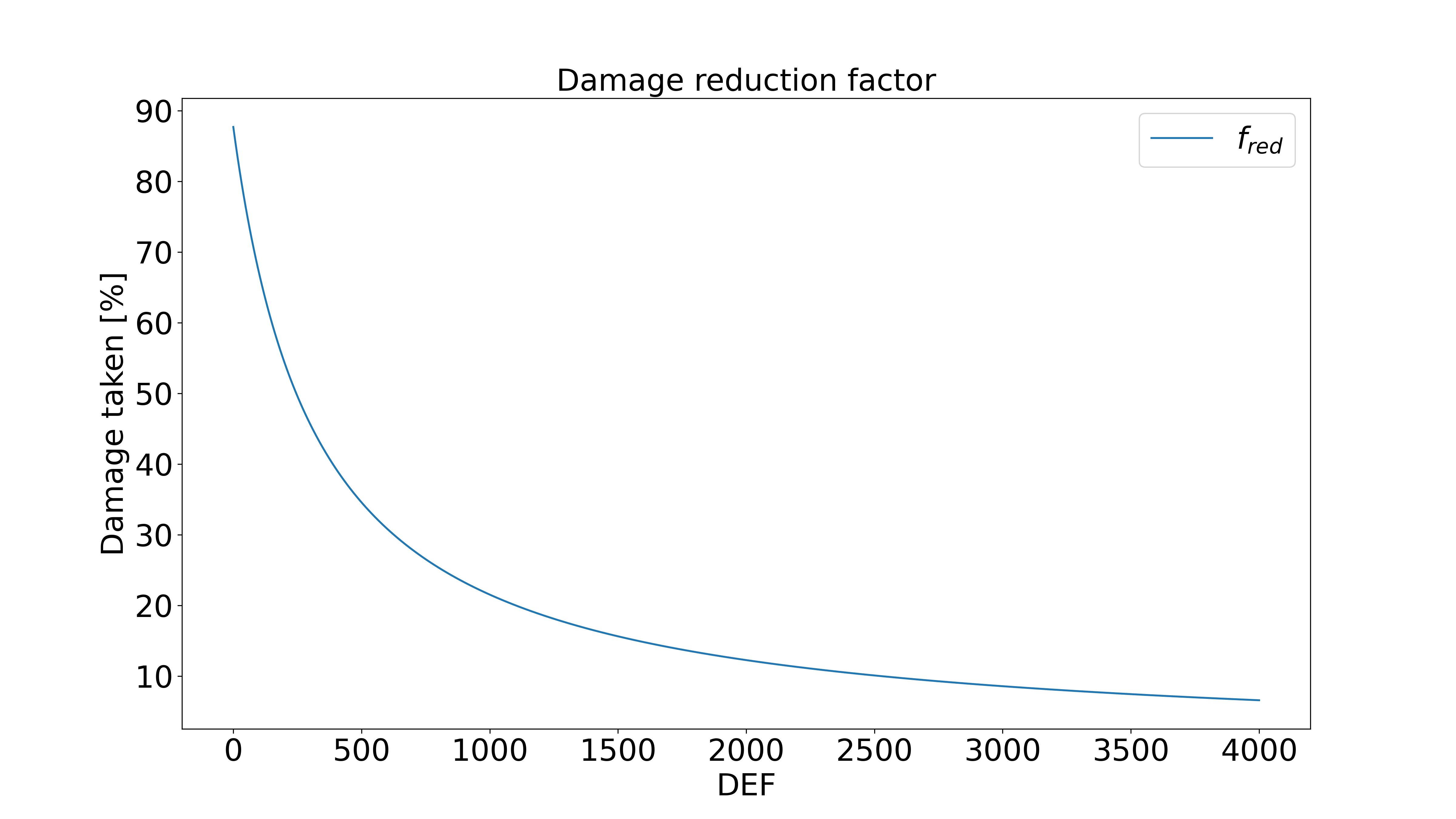# Damage Calculations¶

## General¶

The general formula for the damage of any skill is

\text{Inflicted DMG} = \left(\sum_i S_i \cdot M_i\right)\cdot f_{crit}\cdot f_{def}\cdot f_{fluct}\cdot\prod_j f_{spec,j}

That's a lot of stuff going on so let us break it down:

• $\sum_i S_i \cdot M_i$ is the sum of the stats $S_i$ the skill scales on and their respective multipliers $M_i$. However most skills only scale on one single stat so most of the time it's not a sum but just $stat \cdot multiplier$.
• $f_{crit}$ is the factor increasing your damage when you land a critical hit.
• $f_{def}$ is the damage reduction factor by the enemy's defence. See damage reduction formula for details.
• $f_{fluct}$ is a factor accounting for built-in random $\sim \pm 5\%$ fluctuations of the damage of most skills. (Ignore this when calculating your damage (read: set to 1))
• $\prod_j f_{spec,j}$ is the product of all special damage multipliers $f_{spec,j}$ that apply. For example brand debuff, damage reduction passives, artifacts, damage increase based on number of debuffs, etc.

In the following we will take a detailed look at each of those factors and provide some examples.

## Damage Reduction by Defence Formula¶

The defence of a monster determines by how much the damage that monster will take is being reduced. The formula for the part of the damage that you will take is

f_{def} = \frac{1000}{1140 + 3.5 \cdot DEF}Attention

Ignore DEF skills are still subject to this factor. They just work with $DEF = 0$ so you end up with $f_{def} = \frac{1000}{1140} \approx 87.72\%$. Keep this in mind when calculating your Lushen's damage.

## Damage Types¶

Some skills behave in a special way. Those skills can be put into categories which get their own names. This is similar to how other games might have things like "magical damage" and "physical damage" yet not quite the same because in Summoners War there is no such differentiation, damage types here merely affect the damage calculation.

### True Damage¶

True damage still fluctuates but is not subject to damage reduction by defence ($f_{def} = 1$). The only thing currently in the game dealing true damage are bombs.

### Fixed Damage¶

Fixed damage deals exactly like the damage you calculate, it is neither subject to damage reduction by defence nor the random fluctuation of normal damage skills ($f_{def} = f_{fluct} = 1$). Examples of fixed damage are Skogul's S2, Ramagos' S3, or Arnold's S3.

### Pure Damage¶

Pure damage is pretty much like fixed damage but unlike that it does not get influenced by damage reducing passives or the brand debuff or anything else. Continuous Damage and the first part of Akroma's and Daphnis' S3 are examples of pure damage.

## Hit Types¶

There are four different types of hits an attack can have: Critical Hit, Crushing Hit, Glancing Hit, and Normal Hit.

The order in which the game evaluates as what kind of hit a hit lands is as follows:

1. Check for glancing hit

2. Check for critical hit

3. Check for crushing hit

If none of those checks are passed, then it is a normal hit.

### Critical Hit¶

• Base Critical Rate is 15%.

### Crushing Hit¶

• Deals 30% increased damage

### Glancing Hit¶

• Base chance to glance is 0%.

• If attacking while having Attribute Disadvantage, the chance to glance increases by 50%.

• Deals 30% decreased damage, decreased additionally by 16% if the attacker has Attribute Disadvantage.

• Debuffs and ATB reduction can not happen on Glancing Hits.

### Normal Hit¶

• Set $f_{crit} = 1 + skillups$. Please see skillups for the reason why.